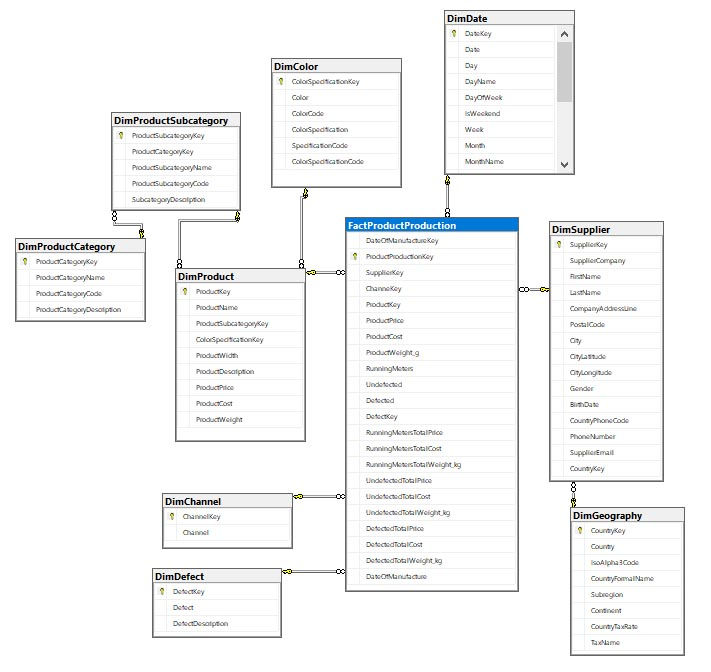top of page
Search
• Marek Vavrovic

# Huffner Textile - Data Warehouse Design

-sql database backup

-SQL Server Database Project

HuffnerTextileDW
.zip

### Types of Data Warehouse Schema

STAR SCHEMA

The simplest and the most widely used dimensional model is a star schema. It has the fact table at the center and the dimensions surrounding it. It can also be described as a parent-child table design. The fact table is the parent while the dimensions are the children. But since it’s so simple, there are no grandchildren.

Common characteristics of star schema include:

1. Fact table is at the center containing dimension keys (foreign keys) and measures.

2. Primary keys in dimension tables are foreign keys in the fact table.

3. No dimension table references another dimension table. They are denormalized.

1. Simpler queries because of the uncomplicated design.

2. Easily maintained.

3. Faster access to records because of the denormalized dimension table design.

SNOWFLAKE SCHEMA

In a snowflake schema, dimension tables are normalized. The physical structure resembles a snowflake shape. Compared to a parent-child design, snowflake schemas can have grandchildren.

Common characteristics of snowflake schema include:

1. Fact table is also at the center, like the star schema.

2. Fact table references first-level dimension tables.

3. Dimension table can reference another dimension table. This design is normalized.

1. More flexible to changes in structure.

2. Less disk space because of normalized dimension tables.

Partitioned table

To create a partitioned table, we'll need to first create a partition function and partition scheme.

CREATE PARTITION FUNCTION Year_PartFunc(int) --data type

AS RANGE RIGHT

FOR VALUES ('20110101','20120101','20130101','20140101','20150101','20160101','20170101','20180101','20190101');

GO

All data before 20110101 will go to PRIMARY filegroup

CREATE PARTITION SCHEME Year_PartScheme

AS PARTITION Year_PartFunc

TO ([PRIMARY],FG2011, FG2012,FG2013,FG2014,FG2015,FG2016,FG2017,FG2018,FG2019);

go

Creating Data Warehouse Database

USE [master]

GO

--database needs to be partitioned before the data are loaded.

CREATE DATABASE [HuffnerTextileDW]

CONTAINMENT = NONE

ON PRIMARY

( NAME = N'HuffnerTextileDW', FILENAME = N'C:\Program Files\Microsoft SQL Server\MSSQL15.MSSQLSERVER\MSSQL\DATA\HuffnerTextileDW.mdf' , SIZE = 532480KB , MAXSIZE = UNLIMITED, FILEGROWTH = 0),

FILEGROUP [FG2011]

( NAME = N'2011Data', FILENAME = N'C:\Program Files\Microsoft SQL Server\MSSQL15.MSSQLSERVER\MSSQL\DATA\2011Data.ndf' , SIZE = 8192KB , MAXSIZE = UNLIMITED, FILEGROWTH = 0),

FILEGROUP [FG2012]

( NAME = N'2012Data', FILENAME = N'C:\Program Files\Microsoft SQL Server\MSSQL15.MSSQLSERVER\MSSQL\DATA\2012Data.ndf' , SIZE = 38464KB , MAXSIZE = UNLIMITED, FILEGROWTH = 0),

FILEGROUP [FG2013]

( NAME = N'2013Data', FILENAME = N'C:\Program Files\Microsoft SQL Server\MSSQL15.MSSQLSERVER\MSSQL\DATA\2013Data.ndf' , SIZE = 46272KB , MAXSIZE = UNLIMITED, FILEGROWTH = 0),

FILEGROUP [FG2014]

( NAME = N'2014Data', FILENAME = N'C:\Program Files\Microsoft SQL Server\MSSQL15.MSSQLSERVER\MSSQL\DATA\2014Data.ndf' , SIZE = 46272KB , MAXSIZE = UNLIMITED, FILEGROWTH = 0),

FILEGROUP [FG2015]

( NAME = N'2015Data', FILENAME = N'C:\Program Files\Microsoft SQL Server\MSSQL15.MSSQLSERVER\MSSQL\DATA\2015Data.ndf' , SIZE = 56064KB , MAXSIZE = UNLIMITED, FILEGROWTH = 0),

FILEGROUP [FG2016]

( NAME = N'2016Data', FILENAME = N'C:\Program Files\Microsoft SQL Server\MSSQL15.MSSQLSERVER\MSSQL\DATA\2016Data.ndf' , SIZE = 61696KB , MAXSIZE = UNLIMITED, FILEGROWTH = 0),

FILEGROUP [FG2017]

( NAME = N'2017Data', FILENAME = N'C:\Program Files\Microsoft SQL Server\MSSQL15.MSSQLSERVER\MSSQL\DATA\2017Data.ndf' , SIZE = 67904KB , MAXSIZE = UNLIMITED, FILEGROWTH = 0),

FILEGROUP [FG2018]

( NAME = N'2018Data', FILENAME = N'C:\Program Files\Microsoft SQL Server\MSSQL15.MSSQLSERVER\MSSQL\DATA\2018Data.ndf' , SIZE = 8192KB , MAXSIZE = UNLIMITED, FILEGROWTH = 0),

FILEGROUP [FG2019]

( NAME = N'2019Data', FILENAME = N'C:\Program Files\Microsoft SQL Server\MSSQL15.MSSQLSERVER\MSSQL\DATA\2019Data.ndf' , SIZE = 8192KB , MAXSIZE = UNLIMITED, FILEGROWTH = 0),

FILEGROUP [INDEXES]

( NAME = N'Indexes', FILENAME = N'C:\Program Files\Microsoft SQL Server\MSSQL15.MSSQLSERVER\MSSQL\DATA\Indexes.ndf' , SIZE = 8192KB , MAXSIZE = UNLIMITED, FILEGROWTH = 0)

LOG ON

( NAME = N'HuffnerTextileDW_log', FILENAME = N'C:\Program Files\Microsoft SQL Server\MSSQL15.MSSQLSERVER\MSSQL\DATA\HuffnerTextileDW_log.ldf' , SIZE = 10029504KB , MAXSIZE = 2048GB , FILEGROWTH = 0)

WITH CATALOG_COLLATION = DATABASE_DEFAULT

GO

Creating dimensions and fact tables.

Database schemas.

There are seven schemas in the HuffnerTextileDW

SuppliersProductionProductionDefectStockInventoryStockMovementsSubDepotClient

For reporting purposes, it is better to join DimGeography with DimWarehouse or DimClient, DimSubDepotEmployee because there will be an inactive relationship between the Fact table and DimGeography when the model is left like this. What means that you won't be able to create the measures unless you do not use USERELATIONSHIP function.